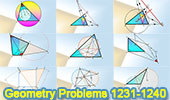# Geometry Problems 1231-1240. Level: High School, CollegeGeometry Problems 1231 - 1240Geometry Problem 1231 Triangle, Orthocenter, Incenter, Circumcenter, Angle Bisector, Center, Circle. Geometry Problem 1232 Triangle, Altitudes, Orthic Triangle, Collinearity, Orthic Axis. Geometry Problem 1233 Triangle, Euler Line, Orthic Axis, Perpendicular, 90 Degrees, Orthic Triangle. Geometry Problem 1234 Quadrilateral, 60 Degrees, Midpoint, Congruence, Cyclic Quadrilateral, Concyclic Points. Geometry Problem 1235 Triangle, Cevians, Concurrency, Circle, Circumcircle. Geometry Problem 1236 Intersecting Circles, Secant, Concyclic Points. Geometry Problem 1237 IMO 2016, Problem 1, Triangle, Congruence, Parallel Lines, Midpoint, Concurrency. Geometry Problem 1238 Square, Equilateral Triangle, Metric Relations. Mobile Apps. Geometry Problem 1239 Intersecting Circles, Secant, Concyclic Points, Congruence. Mobile Apps Geometry Problem 1240 Triangle, Circle, Diameter, Circumcircle, Circumcenter, Perpendicular. Mobile Apps.

Home | Sitemap | Search | Geometry | Problems | Visual Index | 10 ProblemsEmail | Post a comment | by Antonio Gutierrez
Last updated: Feb 1, 2017